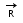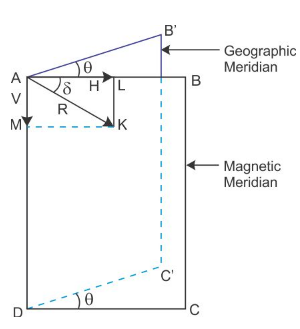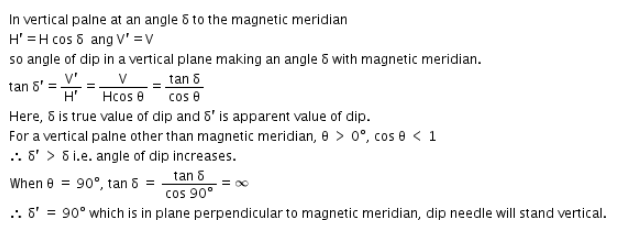# How does a dip circle measures the dip angle at a place?

How does a dip circle measures the dip angle at a place?
also, explain this statement: the dip angle in a vertical plane perpendicular to magnetic meridian will 90 degrees. why?Dip circle use to measure the dip angle.
To use the dip circle: (follow the given image)
(i) Adjust the leveling screw till the base is horizontal and V inside the box is vertical
(ii) Rotate the box B till the ends of magnetic needle NS read 90° - 90° on the vertical scale.
The needle is thins position is aligned with the vertical component of earth’s filed. The horizontal component of earth’s field being perpendicular to this plane does not affect the needle.
The reading of the vernier V’ is noted on horizontal circular scale C.
(iii) Rotate the box exactly through 90° from this position. The plane of vertical scale V is now in magnetic meridian. The total field strength R of earth’s magnetic field is in the same plane and hence magnetic needle rests along. Angle whichmakes the horizontal direction in magnetic meridian is the angle of dip. As horizontal line is marked 0 – 0, the reading of the ends magnetic needle on the vertical scale give us directly the angle of dip (δ)
At different places on the surface of earth, δ is different.